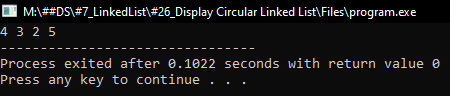# Circular Linked List in C

## Circular Linked List in C Language with Examples:

In this article, I am going to discuss How to Create and Display Circular Linked Lists in C Programming Language with Examples. Please read our previous article, where we discussed How to Check Whether a Linked List is Linear or Not in C Language with Examples.

##### What are the methods to represent a circular linked list?

There are two methods. I will show you both the methods for representing a circular linked list. Let us discuss the first method.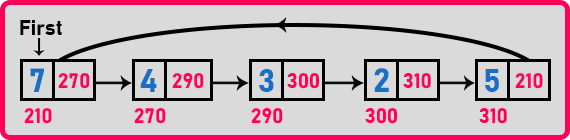We have an example here. This is a circular linked list. So, what is a circular linked list? With respect to a linear linked list, linear means the last node should point to null. In this linked list the last node is pointing to the first node. Then it is a circular linked list. In other words, the nodes are circularly connected.

So, the collection of nodes when they are circularly connected then which one is the first node? So, there is no first node. For that, we usually use the term ‘head’ which is the pointer name for one of the nodes. So, there is no first node or last node. Here ‘head’ is more stable than calling it ‘first’.

##### What is the benefit of a circular linked list?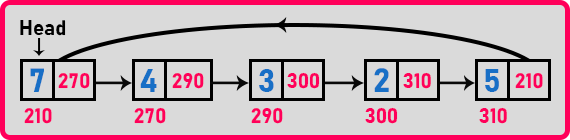We can traverse the nodes. Suppose you have started from the first node and you can go to the next node and we can go back to the head where the links are circularly connected. So, we can traverse this linked list circularly.

In our computer system or in our mobile apps, in many places, we find things that are lists or collections which are handled circularly. If we take the example of a contact listing on your mobile phone, suppose you are scrolling up in the contacts and you have reached the last contact that is starting with the ‘Z’. Then again on some mobile phones or in some apps if you scroll up again the contacts with ‘A’ will start again.

So, it means we are able to access the list of contacts circularly. So just we have added one extra feature to the linked list that we have been studying so far. We’ve been learning about a singly linked list now this is a singly and circular linked list.

##### Where to use the circular linked list?

If you have only one direction access and you also want circular access then you can go for it. Now when we talk about circular linked lists, we don’t have to study much because what you can do on a linear linked list, is the same thing you can do here also. Few important things we will discuss. Now let us see more about the circular linked lists.See there are five nodes in this linked list. Suppose there is only one node in the link list. Then it should point on itself then it is circular.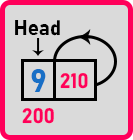If there are zero nodes or no nodes in the linked list then ‘Head’ will point to null. Now one problem is supposed we have a linear linked list. If there are no nodes in the linear linked list then the ‘first’ pointer will point to null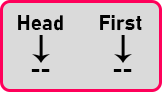So, if there are no nodes in a circular linked list and no nodes in a linear linked list, both are null so how do you prove that this is circular? There is no way to show that this is circular. From the name, we cannot say that this is circular as both are null.

So, there is an argument that circular linked lists cannot be NULL, if it is empty also it should be circular. In the above example, ‘Head’ is empty as there are no nodes but it should be circular. So, to support this argument there is one more representation we will draw and show you.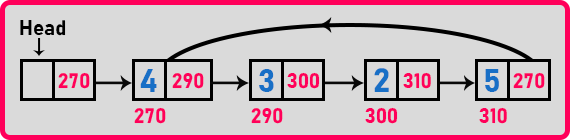This is the second representation of the circular linked list. Based on the argument that if it is empty also should be circular. So, to support this argument we have this representation. Rest is the choice for programmer or developer to which one they want to implement circular linked list either 1st or 2nd representation.

Here, only 4 nodes are present in the above circular linked list, and the last node is pointing to the first node of the linked list which is ‘4’. And these nodes are circularly connected. The node where ‘Head’ is pointing is empty. So, what is the purpose of this node? This is acting as ‘Head’. It is pointing to one of the nodes in the collection of circularly connected nodes. So that we can access the circular linked list. There is no data in the ‘Head’ node. It will not use to store the data. After the head node then we will start storing the data. So, there are only 4 nodes in this linked list, not 5.

How it looks if there are no nodes at all? Let see,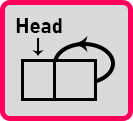Now it is empty and it is circular. If you are starting from the head then you will reach on the head only.

So, we have studied two representations:

###### 1st Representation of Circular Linked List:###### 2nd Representation of Circular Linked List:Which representation should be followed? That is your choice. In your application or program, you can use any of the representations. There is not a big change in that we are introducing an empty node and making it circular even if it is empty. Commonly used as a linked list even in the books you will find the first representation. If you follow the second representation also the procedure will be the same as that linked list.

##### How to Display Circular Linked List in C Language:

Now, we will learn about how to display a circular linked list. We already know how to display a linear linked list. The procedure will be the same definitely but the difference in the linear linked list is the last node was null so we are stopping there.But now in the circular linked list, if we start from the ‘Head’ node then if you go on accessing further nodes then you will be reaching back on the same node. So, we have to stop when we are reaching the starting point again. Then the list has finished. Let us see how we can do this.

##### Procedure to Display Circular Linked List:

Let us take a pointer ‘p’ which is pointing to the head’s node.Here we will print the p’s data and move ‘p’ to the next node.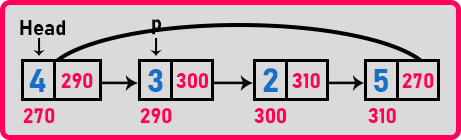Here again, print the p’s data and move ‘p’ to the next node.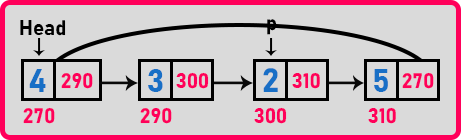Here ‘2’ will be displayed and ‘p’ will move to the next node.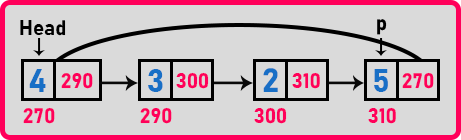Now ‘5’ will be displayed and ‘p’ will move to the next node.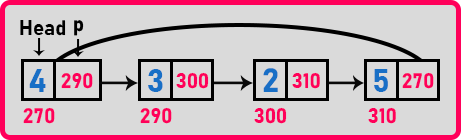Now, ‘p’ is again pointing to the head’s node so here we will not print any value. We have to stop when ‘p’ reaches the head once again. We will write a condition that will continue if ‘p’ is not equal to the head. But the problem is when we start, ‘p’ is already equal to ‘head’. It will not print the linked list as ‘p’ is equal to the head. This cannot be done by using a while loop.

We have to print the linked list using a do-while loop because it will run at least one time where while first checking the condition and then executing the statements if the condition is true. Pseudocode by the do-while loop is,

```void Display(Node *p){
do{
printf("%d", p->data);
p = p->next;
}```

How to know if it’s the first time or the second time? So, for that, we have to take some variable flag. We will write the flag as,

void Display(Node *p){
int flag = 0;
———
}
}

Here flag value ‘0’ means ‘p’ is pointing to the head first time and if flag value ‘1’ means ‘p’ is pointing to the head the second time. With the help of ‘0’ and ‘1’, we can identify whether it is the first time or the second time ‘p’ is pointing at the head.

Inside the if-block, we will write the print statement and then call the function again. And once control enters inside if-block then the flag should become 1.

```void Display(Node *p){
int flag = 0;
if (p != head || flag == 0){
flag = 1;
printf(“%d”, p->data);
Display (p->next);
}
}```

Now let us trace this function. The first ‘p’ is on the head,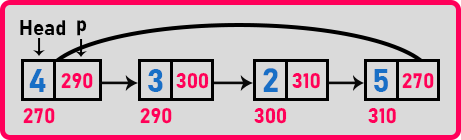Condition is ‘if (p != head || flag == 0)’, here p is on the head so the first condition is false then the flag is 0 as it is or operator and when any of the conditions is true then the whole condition will true so then it will enter in the if-block and then modify flag to ‘1’ and print ‘4’. Then call the function again with the argument ‘p->next’.Now this time ‘p’ is not equal to the head so it will not check for the flag and enter in the if-block. Then modify the flag to ‘1’ and print ‘3’ then call the function again with ‘p->next’.Here again ‘p’ is not equal to the head so it will enter in the if-block. So, it modifies the flag to ‘1’ and prints ‘2’ then calls the function with ‘p->next’.Now again ‘p’ is not equal to head so it will enter in the if-block. So again, it modifies the flag to ‘1’ and prints ‘5’ then calls the function with ‘p->next’.Here ‘p’ again points on the head. There the condition will not satisfy as ‘p’ is on the head and the flag is not zero. So, both conditions are false. It will exit the call of the function. When the call exits then we have to write a statement that is ‘flag = 0’.

```void Display(Node *p){
int flag = 0;
if (p != head || flag == 0){
flag = 1;
printf(“%d”, p->data);
Display (p->next);
}
flag = 0;
}
```

Now one important thing is, in every recursive call the new ‘flag’ variable will be created in the stack memory. Every time the flag becomes zero. It will not have the same flag. What we want is for all recursive calls there is only one flag. Then we can declare the flag outside the function as a global variable or we can make it static inside the function so that only one copy exists throughout the program. For the above-linked list, our recursive function will be called 5 times.

##### Pseudo code to display circular linked list recursively:
```void Display(Node *p){
static int flag = 0;
if (p != head || flag == 0){
flag = 1;
printf(“%d”, p->data);
Display (p->next);
}
flag = 0;
}
```

Now let us write the complete program to display a circular linked list.

##### Program to display Circular Linked List using C Language
```#include <stdio.h>
#include <stdlib.h>

struct Node
{
int data;
struct Node *next;

void Create(int A[], int n)
{
int i;
struct Node *t, *last;
Head = (struct Node *) malloc(sizeof(struct Node));

for (i = 1; i < n; i++)
{
t = (struct Node *) malloc (sizeof (struct Node));
t->data = A[i];
t->next = last->next;
last->next = t;
last = t;
}
}

void Display(struct Node *h)
{
do
{
printf ("%d ", h->data);
h = h->next;
}
printf ("\n");
}

void RDisplay(struct Node *h)
{
static int flag = 0;
if (h != Head || flag == 0)
{
flag = 1;
printf ("%d ", h->data);
RDisplay(h->next);
}
flag = 0;
}

int main()
{
int A[] = { 4, 3, 2, 5 };
Create(A, 4);